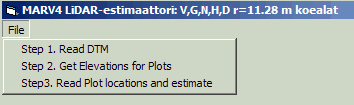Program for computing the total stem volume (V), basal area (G), stem number (N), basal area median height (HgM), Basal area median diameter (DgM) using LiDAR data The program operates on the 2004, sparse LiDAR, and estimates the 5 variables for circular plots (r=11.28 m). The IDs and XY positions of the plots are given in an ASCII file C:\data\marv4_alat.txt. You can change values in this file, and study, for example what happens if the XY-grid moves 1 m to North. The DTM is needed for computing the above ground heights for the LiDAR points. It is a 26 MB binary file that has an associated ASCII-header. The ALS2004 1-hactare binary files (*.bin) are needed, and they should be in folder c:\data\als2004\Repros\Bin\. They require storage of 1.12 Gb. Zip-file with Windows executable and source Windows executable. The program execution has three steps:In step one, read the hdr-file for the DTM. In step two, the program calculates terrain elevations for the plots. In step three, the marv4_alat.txt file is read, and the 5 variables are estimated. Output is stored in c:\data\Regression_results.txt. It contains values for plotID, X, Y, Volume [m3/ha], Basal area [m2/ha], Stem number [n/ha], mean height [m], mean diameter [cm]